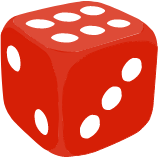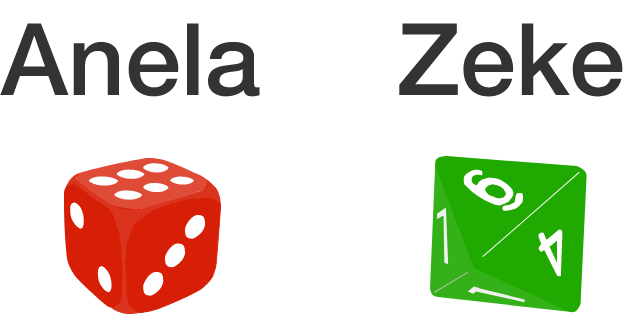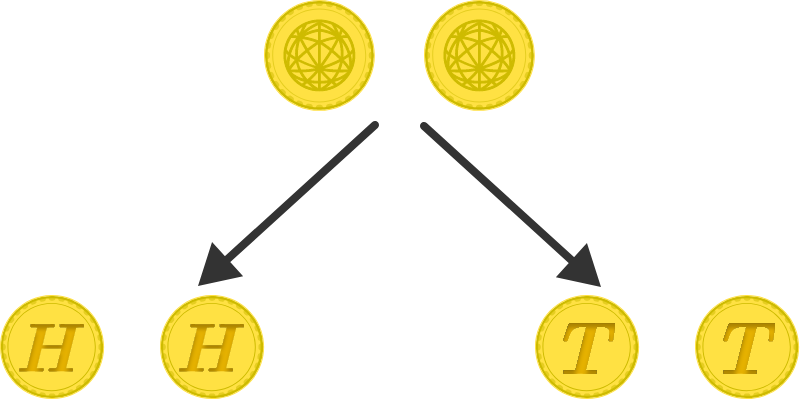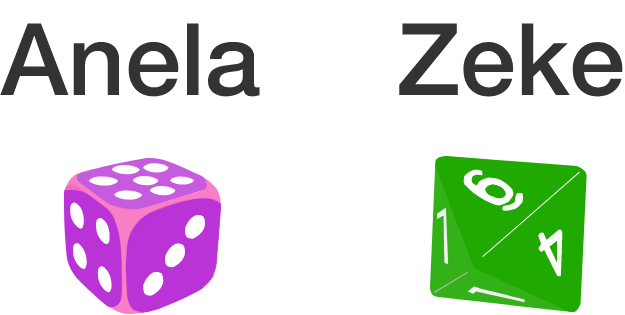### Probability Fundamentals

A probability is the measurement of what chance some specified outcome has for happening. Probabilities are typically either expressed as a percentage, measured from $0\%$ to $100\%,$ or a fraction $($between $0$ to $1).$

For example, if you roll a single standard die, the probability of an even number is exactly $50\%$ or $\frac{1}{2}$ since, of the $6$ possible outcomes for what might be rolled, $3$ are even and $3$ are odd.For the problems in this sequence, your job is to intuit if the event that's specified is less than, more than, or exactly $50\%$ likely to occur. These next five questions are meant only to test your intuition, but you can find the complete explanations if you're curious. We'll move on and introduce how to calculate probabilities numerically in the next two sets of challenges!Anela throws a standard six-sided die $($marked from $1$ to $6)$ while Zeke throws a standard eight-sided die $($marked from $1$ to $8).$

The probability Zeke throws a larger number than Anela is $\text{\_\_\_\_\_\_\_\_\_\_}.$You throw a pair of fair coins. The probability that the result is either both are heads or both are tails is $\text{\_\_\_\_\_\_\_\_\_\_}.$In the region where Cormac lives, it is sunny three-quarters of the days and rains for the rest.

Every day Cormac makes a weather prediction by flipping a fair coin; heads mean predicting sun and tails mean predicting rain.

The probability Cormac will correctly predict the weather this way on a particular day is $\text{\_\_\_\_\_}.$A standard deck with $52$ cards — $26$ red and $26$ black — is shuffled.

The probability that the top card is the same color as the bottom card is $\text{\_\_\_\_\_\_\_\_\_\_}.$Let's modify the problem you saw earlier with Anela and Zeke slightly:

• Zeke still throws a standard eight-sided die $($marked from $1$ to $8).$
• However, Anela now throws a non-standard six-sided die that is marked from $2$ to $7$.

Which of these events are more likely to occur?

• Zeke throws a larger number than Anela.

• Zeke throws a smaller number than Anela.